Search by Topic

Resources tagged with Dice similar to Taking a Die for a Walk:

Filter by: Content type:
Age range:
Challenge level:

There are 35 results

Broad Topics > Mathematics Tools > Dice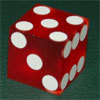Taking a Die for a Walk

Age 5 to 11 Challenge Level:

Investigate the numbers that come up on a die as you roll it in the direction of north, south, east and west, without going over the path it's already made.Dice Stairs

Age 7 to 11 Challenge Level:

Can you make dice stairs using the rules stated? How do you know you have all the possible stairs?Two Dice

Age 5 to 7 Challenge Level:

Find all the numbers that can be made by adding the dots on two dice.Scoring with Dice

Age 7 to 11 Challenge Level:

I throw three dice and get 5, 3 and 2. Add the scores on the three dice. What do you get? Now multiply the scores. What do you notice?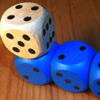The Dice Train

Age 7 to 11 Challenge Level:

This dice train has been made using specific rules. How many different trains can you make?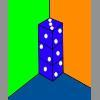Dice in a Corner

Age 7 to 11 Challenge Level:

How could you arrange at least two dice in a stack so that the total of the visible spots is 18?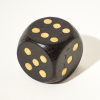Double or Halve?

Age 5 to 7 Challenge Level:

Throw the dice and decide whether to double or halve the number. Will you be the first to reach the target?Round the Dice Decimals 1

Age 7 to 11 Challenge Level:

Use two dice to generate two numbers with one decimal place. What happens when you round these numbers to the nearest whole number?Round the Dice Decimals 2

Age 7 to 11 Challenge Level:

What happens when you round these numbers to the nearest whole number?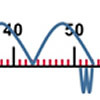Five Steps to 50

Age 5 to 7 Challenge Level:

Use five steps to count forwards or backwards in 1s or 10s to get to 50. What strategies did you use?Dice and Spinner Numbers

Age 7 to 11 Challenge Level:

If you had any number of ordinary dice, what are the possible ways of making their totals 6? What would the product of the dice be each time?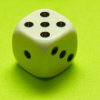A Bit of a Dicey Problem

Age 7 to 11 Challenge Level:

When you throw two regular, six-faced dice you have more chance of getting one particular result than any other. What result would that be? Why is this?Roll These Dice

Age 7 to 11 Challenge Level:

Roll two red dice and a green dice. Add the two numbers on the red dice and take away the number on the green. What are all the different possible answers?Round the Two Dice

Age 5 to 7 Challenge Level:

This activity focuses on rounding to the nearest 10.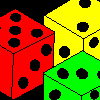Age 7 to 11 Challenge Level:

Three dice are placed in a row. Find a way to turn each one so that the three numbers on top of the dice total the same as the three numbers on the front of the dice. Can you find all the ways to do. . . .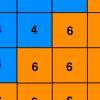Six Ten Total

Age 7 to 11 Challenge Level:

This challenge combines addition, multiplication, perseverance and even proof.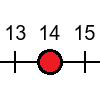Tug of War

Age 5 to 7 Challenge Level:

Can you use the numbers on the dice to reach your end of the number line before your partner beats you?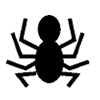KS2 Teacher Playing with Dice Collection

Age 7 to 11 Challenge Level: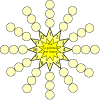The Twelve Pointed Star Game

Age 7 to 11 Challenge Level:

Have a go at this game which involves throwing two dice and adding their totals. Where should you place your counters to be more likely to win?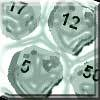Asteroid Blast

Age 7 to 11 Challenge Level:

A game for 2 people. Use your skills of addition, subtraction, multiplication and division to blast the asteroids.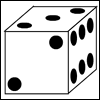Right or Left?

Age 7 to 11 Challenge Level:

Which of these dice are right-handed and which are left-handed?Three Dice

Age 7 to 11 Challenge Level:

Investigate the sum of the numbers on the top and bottom faces of a line of three dice. What do you notice?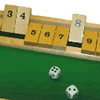Shut the Box for Two

Age 5 to 7 Challenge Level:

Shut the Box game for an adult and child. Can you turn over the cards which match the numbers on the dice?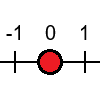Tug Harder!

Age 7 to 11 Challenge Level:

In this game, you can add, subtract, multiply or divide the numbers on the dice. Which will you do so that you get to the end of the number line first?First Connect Three

Age 7 to 14 Challenge Level:

The idea of this game is to add or subtract the two numbers on the dice and cover the result on the grid, trying to get a line of three. Are there some numbers that are good to aim for?Round the Four Dice

Age 7 to 11 Challenge Level:

This activity involves rounding four-digit numbers to the nearest thousand.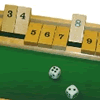Shut the Box

Age 5 to 7 Challenge Level:

An old game but lots of arithmetic!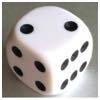Roll over the Dice

Age 7 to 11 Challenge Level:

Watch this video to see how to roll the dice. Now it's your turn! What do you notice about the dice numbers you have recorded?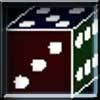The Path of the Dice

Age 7 to 11 Challenge Level:

A game for 1 person. Can you work out how the dice must be rolled from the start position to the finish? Play on line.Odds or Sixes?

Age 7 to 11 Challenge Level:

Use the interactivity or play this dice game yourself. How could you make it fair?Game of PIG - Ones

Age 5 to 7 Challenge Level:

Play a dice game of chancePath of Discovery Series: 2. First Steps

Age 5 to 7

This article takes a closer look at some of the toys and games that can enhance a child's mathematical learning.Dice, Routes and Pathways

Age 5 to 14

This article for teachers discusses examples of problems in which there is no obvious method but in which children can be encouraged to think deeply about the context and extend their ability to. . . .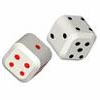Tricky Track

Age 7 to 11 Challenge Level:

In this game you throw two dice and find their total, then move the appropriate counter to the right. Which counter reaches the purple box first?Spinners Environment

Age 5 to 18 Challenge Level:

A tool for generating random integers.